Courses

# Digital Electronics MSQ

## 10 Questions MCQ Test Topic wise Tests for IIT JAM Physics | Digital Electronics MSQ

Description
This mock test of Digital Electronics MSQ for Physics helps you for every Physics entrance exam. This contains 10 Multiple Choice Questions for Physics Digital Electronics MSQ (mcq) to study with solutions a complete question bank. The solved questions answers in this Digital Electronics MSQ quiz give you a good mix of easy questions and tough questions. Physics students definitely take this Digital Electronics MSQ exercise for a better result in the exam. You can find other Digital Electronics MSQ extra questions, long questions & short questions for Physics on EduRev as well by searching above.
*Multiple options can be correct
QUESTION: 1

### Which of the following gives the Boolean expression for Y of the 3-variable truth table Incorrect given below? Truth table.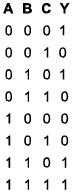Solution:

The above truth table is satisfied by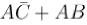further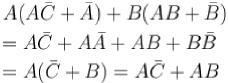The correct answers are: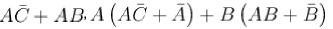*Multiple options can be correct
QUESTION: 2

### Which of the following sets of values given below satisfy the Boolean relation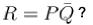Solution:

The realization of the algebraic expression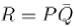in terms of logic gate is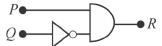Truth table.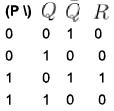Hence, (a), (c), (d)
The correct answers are: P = 0, Q = 0, R = 0, P = 1, Q = 0, R = 1, P = 1, Q = 1, R = 0

*Multiple options can be correct
QUESTION: 3

### The circuit shown in the figure can be used as :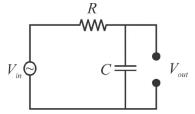Solution: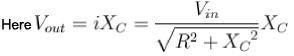Hence, it is an integrator and a low-pass filter.
The correct answers are: Integrator, Low-pass filter

*Multiple options can be correct
QUESTION: 4

A pulse is applied to each input of an exclusive OR gate. One pulse goes HIGH at t = 0 and goes back LOW at t = 1 ms. The other pulse goes HIGH at = 0.8 ms and goes back LOW at t = 3 ms. The output pulse can be described as follows :

Solution:

Output is HIGH when only one of the input is high.
The correct answers are: It goes HIGH at t = 0 and back LOW at t = 0.8 ms, It goes HIGH at t = 1 ms and back LOW at t = 3 ms

*Multiple options can be correct
QUESTION: 5

If the output of a logic gate is 0 only when all its inputs are at logic 1, then which of the following are/is not the corresponding gate?

Solution:

Truth table is,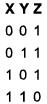This is truth table for NAND gate.
NOR,
AND,
NOT

*Multiple options can be correct
QUESTION: 6

The following circuit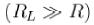does not performs the operation of.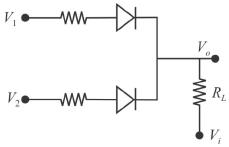Solution:

If either V1 or V2 is high, we get a high in output, hence it each like an OR gate.
The correct answers are: NAND gate for a negative logic system, AND gate for a positive logic system, AND gate for a negative logic system

*Multiple options can be correct
QUESTION: 7

Which of the following options is false for a two input XOR gate?

Solution: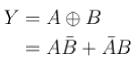The truth table for XOR gate is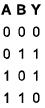(b), (c), (d).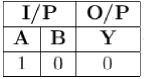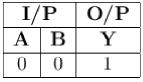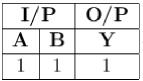*Multiple options can be correct
QUESTION: 8

Inputs given to a logic gate are A and B and its output is X. If A = 1, B = 0, then X = 1. What type of gate this could be?

Solution: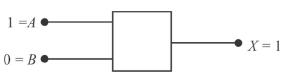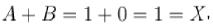clearly it follows OR gate.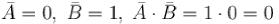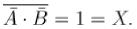Thus, it also follows NAND gate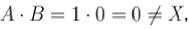it is an AND gate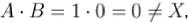it is not a NOR gate.
Therefore, the correct options are (b, c)
The correct answers are: NAND, OR

*Multiple options can be correct
QUESTION: 9

The diagram of a logic circuit is given below. Which of the following represents the output F?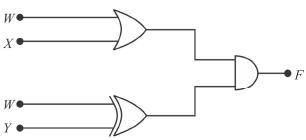Solution: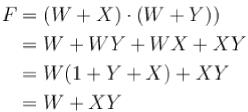The correct answers are: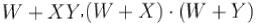*Multiple options can be correct
QUESTION: 10

A pulse is applied to each input of a 2 input NAND gate, one pulse goes high at t = 0 and goes back LOW at t = 1 ms. The other pulse goes high at f = 0.8 ms and goes Mark 0.00 out of 2.00 back LOW at t = 3 ms. The output pulse.

Solution:

A NAND gate is given by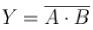Truth table is.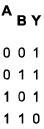It is low when both input signals are high otherwise it goes high.
The correct answers are: goes low at t = 0.8 ms, comes back high at t = 1 ms